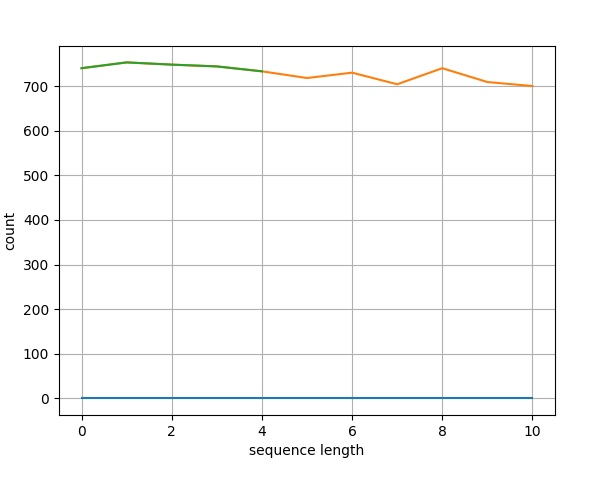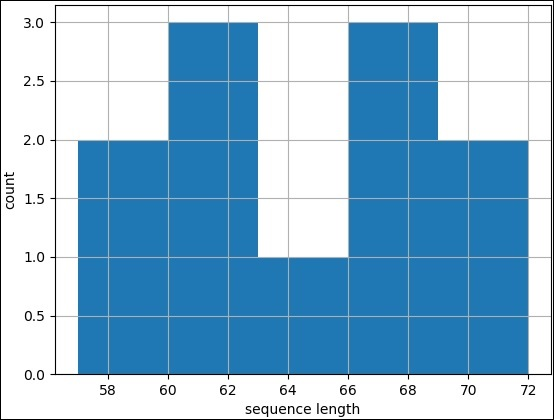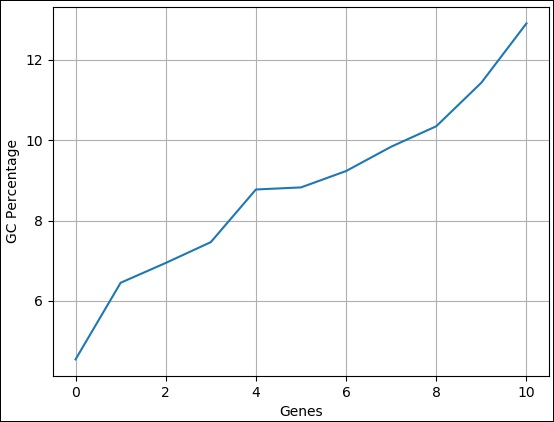# Biopython - Plotting

This chapter explains about how to plot sequences. Before moving to this topic, let us understand the basics of plotting.

## Plotting

Matplotlib is a Python plotting library which produces quality figures in a variety of formats. We can create different types of plots like line chart, histograms, bar chart, pie chart, scatter chart, etc.

pyLab is a module that belongs to the matplotlib which combines the numerical module numpy with the graphical plotting module pyplot.Biopython uses pylab module for plotting sequences. To do this, we need to import the below code −

```import pylab
```

Before importing, we need to install the matplotlib package using pip command with the command given below −

```pip install matplotlib
```

## Sample Input File

Create a sample file named plot.fasta in your Biopython directory and add the following changes −

```>seq0 FQTWEEFSRAAEKLYLADPMKVRVVLKYRHVDGNLCIKVTDDLVCLVYRTDQAQDVKKIEKF
>seq2 EEYQTWEEFARAAEKLYLTDPMKVRVVLKYRHCDGNLCMKVTDDAVCLQYKTDQAQDVKKVEKLHGK
>seq3 MYQVWEEFSRAVEKLYLTDPMKVRVVLKYRHCDGNLCIKVTDNSVCLQYKTDQAQDV
>seq4 EEFSRAVEKLYLTDPMKVRVVLKYRHCDGNLCIKVTDNSVVSYEMRLFGVQKDNFALEHSLL
>seq5 SWEEFAKAAEVLYLEDPMKCRMCTKYRHVDHKLVVKLTDNHTVLKYVTDMAQDVKKIEKLTTLLMR
>seq6 FTNWEEFAKAAERLHSANPEKCRFVTKYNHTKGELVLKLTDDVVCLQYSTNQLQDVKKLEKLSSTLLRSI
>seq7 SWEEFVERSVQLFRGDPNATRYVMKYRHCEGKLVLKVTDDRECLKFKTDQAQDAKKMEKLNNIFF
>seq9 KNWEDFEIAAENMYMANPQNCRYTMKYVHSKGHILLKMSDNVKCVQYRAENMPDLKK
>seq10 FDSWDEFVSKSVELFRNHPDTTRYVVKYRHCEGKLVLKVTDNHECLKFKTDQAQDAKKMEK
```

## Line Plot

Now, let us create a simple line plot for the above fasta file.

Step 1 − Import SeqIO module to read fasta file.

```>>> from Bio import SeqIO
```

Step 2 − Parse the input file.

```>>> records = [len(rec) for rec in SeqIO.parse("plot.fasta", "fasta")]
>>> len(records)
11
>>> max(records)
72
>>> min(records)
57
```

Step 3 − Let us import pylab module.

```>>> import pylab
```

Step 4 − Configure the line chart by assigning x and y axis labels.

```>>> pylab.xlabel("sequence length")
Text(0.5, 0, 'sequence length')

>>> pylab.ylabel("count")
Text(0, 0.5, 'count')
>>>
```

Step 5 − Configure the line chart by setting grid display.

```>>> pylab.grid()
```

Step 6 − Draw simple line chart by calling plot method and supplying records as input.

```>>> pylab.plot(records)
[<matplotlib.lines.Line2D object at 0x10b6869d 0>]
```

Step 7 − Finally save the chart using the below command.

```>>> pylab.savefig("lines.png")
```

### Result

After executing the above command, you could see the following image saved in your Biopython directory.## Histogram Chart

A histogram is used for continuous data, where the bins represent ranges of data. Drawing histogram is same as line chart except pylab.plot. Instead, call hist method of pylab module with records and some custum value for bins (5). The complete coding is as follows −

Step 1 − Import SeqIO module to read fasta file.

```>>> from Bio import SeqIO
```

Step 2 − Parse the input file.

```>>> records = [len(rec) for rec in SeqIO.parse("plot.fasta", "fasta")]
>>> len(records)
11
>>> max(records)
72
>>> min(records)
57
```

Step 3 − Let us import pylab module.

```>>> import pylab
```

Step 4 − Configure the line chart by assigning x and y axis labels.

```>>> pylab.xlabel("sequence length")
Text(0.5, 0, 'sequence length')

>>> pylab.ylabel("count")
Text(0, 0.5, 'count')
>>>
```

Step 5 − Configure the line chart by setting grid display.

```>>> pylab.grid()
```

Step 6 − Draw simple line chart by calling plot method and supplying records as input.

```>>> pylab.hist(records,bins=5)
(array([2., 3., 1., 3., 2.]), array([57., 60., 63., 66., 69., 72.]), <a list
of 5 Patch objects>)
>>>
```

Step 7 − Finally save the chart using the below command.

```>>> pylab.savefig("hist.png")
```

### Result

After executing the above command, you could see the following image saved in your Biopython directory.## GC Percentage in Sequence

GC percentage is one of the commonly used analytic data to compare different sequences. We can do a simple line chart using GC Percentage of a set of sequences and immediately compare it. Here, we can just change the data from sequence length to GC percentage. The complete coding is given below −

Step 1 − Import SeqIO module to read fasta file.

```>>> from Bio import SeqIO
```

Step 2 − Parse the input file.

```>>> from Bio.SeqUtils import GC
>>> gc = sorted(GC(rec.seq) for rec in SeqIO.parse("plot.fasta", "fasta"))
```

Step 3 − Let us import pylab module.

```>>> import pylab
```

Step 4 − Configure the line chart by assigning x and y axis labels.

```>>> pylab.xlabel("Genes")
Text(0.5, 0, 'Genes')

>>> pylab.ylabel("GC Percentage")
Text(0, 0.5, 'GC Percentage')
>>>
```

Step 5 − Configure the line chart by setting grid display.

```>>> pylab.grid()
```

Step 6 − Draw simple line chart by calling plot method and supplying records as input.

```>>> pylab.plot(gc)
[<matplotlib.lines.Line2D object at 0x10b6869d 0>]
```

Step 7 − Finally save the chart using the below command.

```>>> pylab.savefig("gc.png")
```

### Result

After executing the above command, you could see the following image saved in your Biopython directory.